# Digitally controlled potentiometer sets cutoff frequency

## Linear Technology LT1097

Chuck Wojslaw

EDN

The traditional method of controlling the upper cutoff frequency in the basic inverting-amplifier circuit of Figure 1 is to add capacitor C in parallel with R2. The value of C controls the cutoff frequency. R1 and R2 independently establish the magnitude of the circuit gain, which equals R2/R1. If you need a variable cutoff frequency, you use a variable capacitor. However, this approach has two major problems: The circuit does not lend itself to computer control, and few variable capacitors are available with values in the nanofarad region.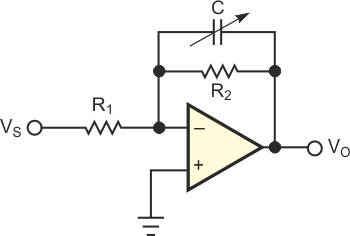Figure 1. Controlling the cutoff frequency using a traditional inverting amplifier circuit has some limitations, such as a limited selection of variable capacitors.

The circuit in Figure 2a is an inverting amplifier that uses a digitally controlled potentiometer and a fixed capacitor as an input Tee network. The magnitude of the gain for this inverting circuit is also R2/R1. However, in this case, R1, C, and the location of the wiper along the resistor array of the potentiometer establish the cutoff frequency. The upper cutoff frequency is programmable because the wiper of the potentiometer is under digital or computer control.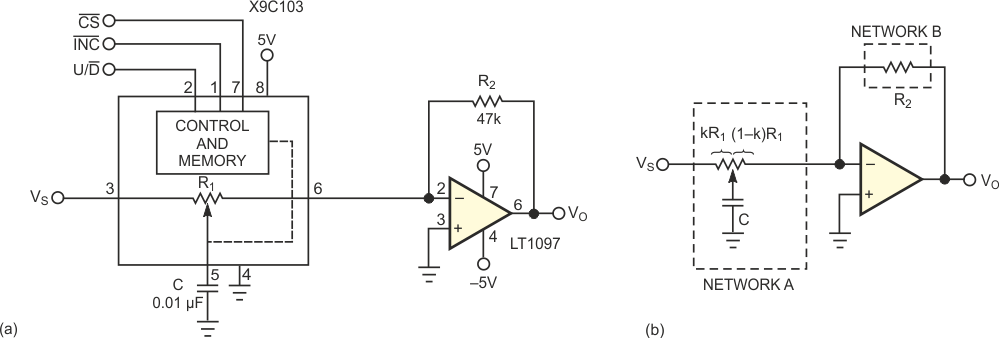Figure 2. An inverting amplifier that uses a digitally controlled potentiometer and a fixed capacitor provides for a programmable cutoff frequency (a). You can analyze the circuit as a two-port network (b).

Several analysis approaches help determine the circuit gain as a function of frequency. One approach is to use y, or admittance, parameters. If you treat networks A and B as two ports (Figure 2b), the ratio of the short-circuit admittance coefficient for the input port, y21A, to y12B for the feedback port produces the following gain expression: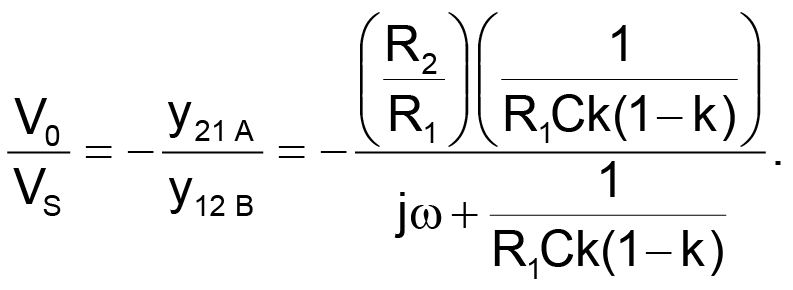In this equation, k is a number that varies from 0 to 1 and reflects the proportionate position of the wiper from one end of the potentiometer (0) to the other end (1).

The circuit's gain expression is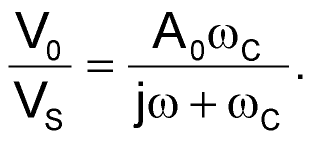This equation has the same form as an equation for an amplifier or lowpass filter with a gain of –R2/R1 and a cutoff frequency of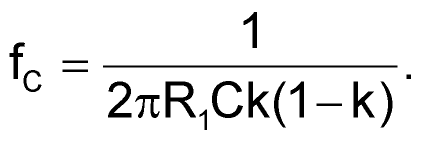As you program the wiper from one end of the potentiometer to the other, k varies from 0 to midscale (1/2) to 1, and the cutoff frequency varies from infinite hertz to a minimum frequency and back to infinite hertz. The minimum frequency is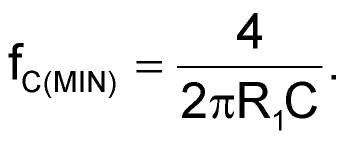For the XDCP family of digitally controlled potentiometers, k can vary from 0 to 1. The number of taps or programmable wiper positions determines the resolution. R1 represents the RTOTAL of the potentiometer. The number of taps varies from 32 to 256, and RTOTAL varies from 1 kΩ to 1 MΩ, depending on the potentiometer. The potentiometer can store a wiper or cutoff-frequency setting in nonvolatile memory, which permits the circuit's cutoff frequency to return to a predetermined value on power-up.

For the circuit in Figure 2a, gain is 4.7, and the cutoff frequency varies from 6.4 kHz to a theoretically infinite hertz. The circuit uses a 10-kΩ potentiometer, the X9C103, which has 100 taps and a three-wire interface. The circuit is useful for audio, control, and signal-processing applications.

EDN